Publicité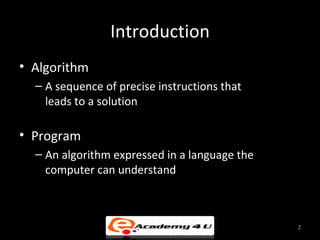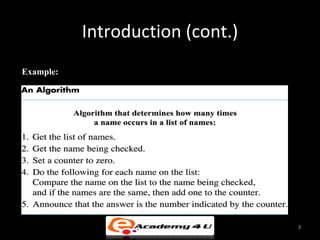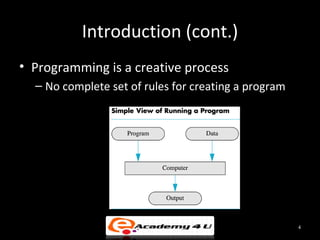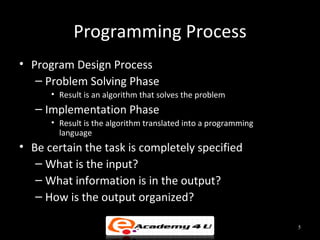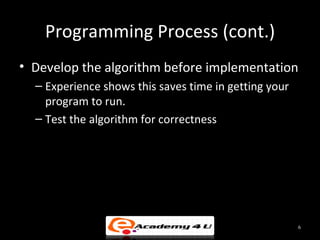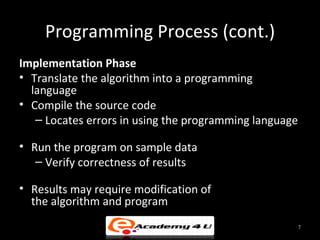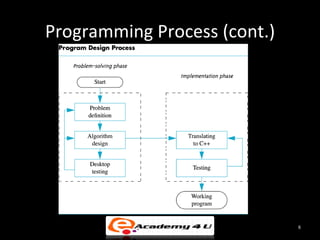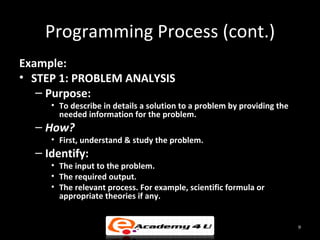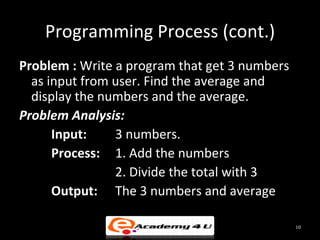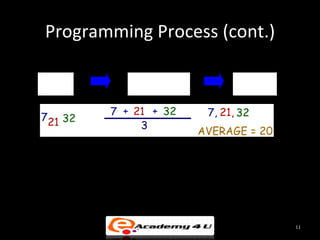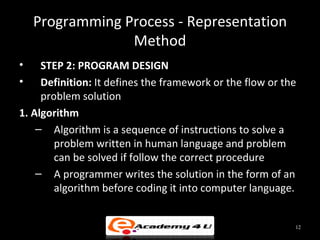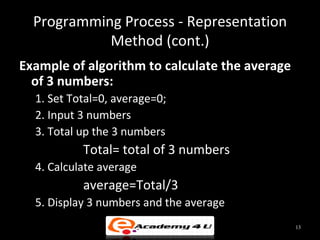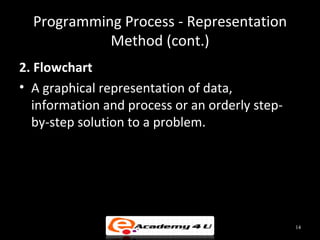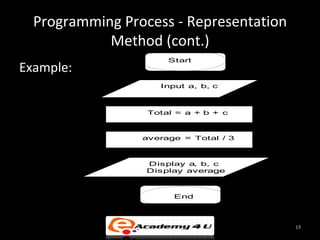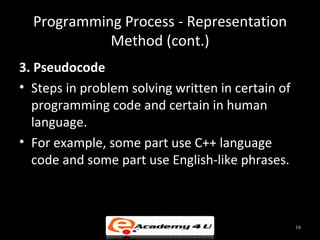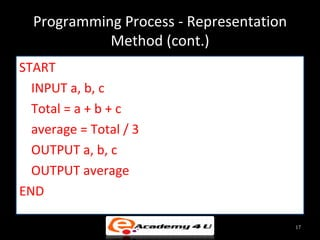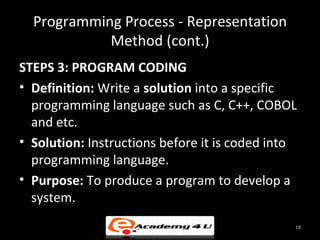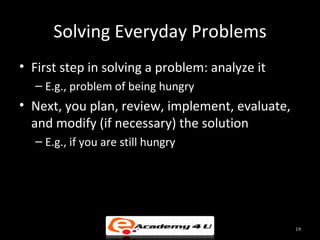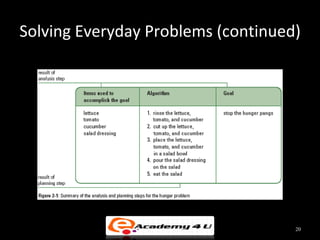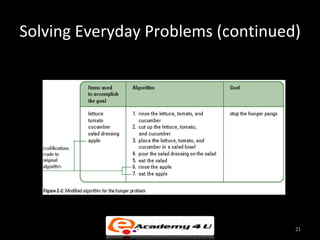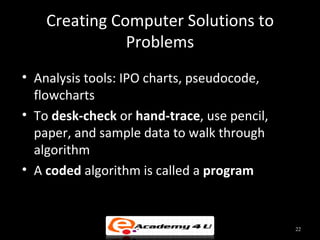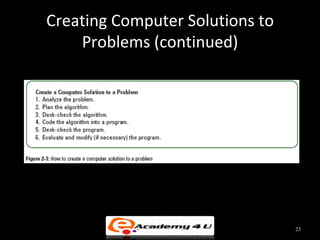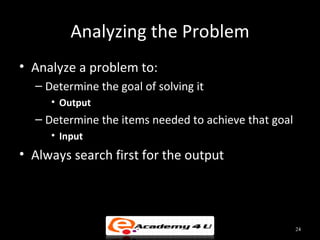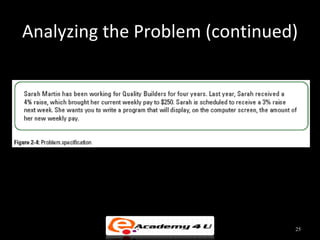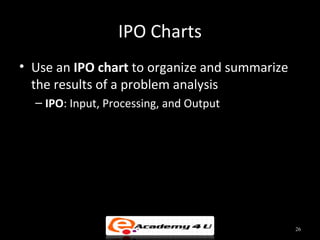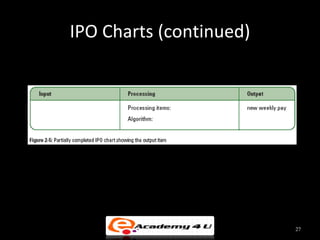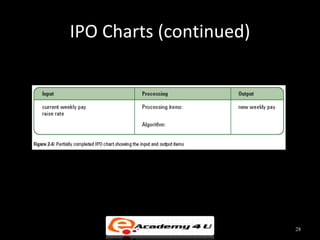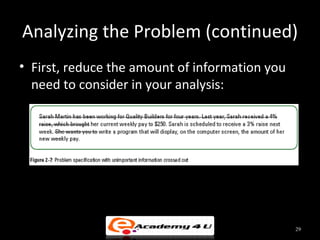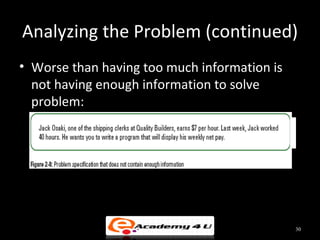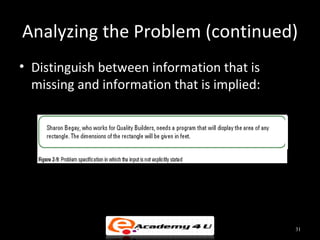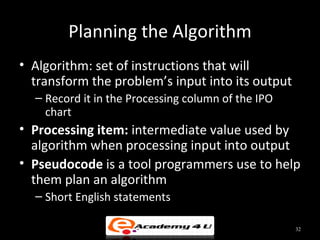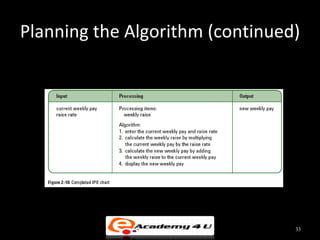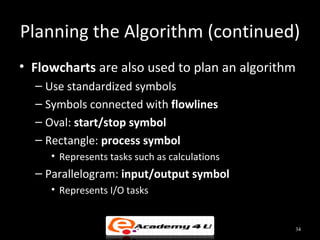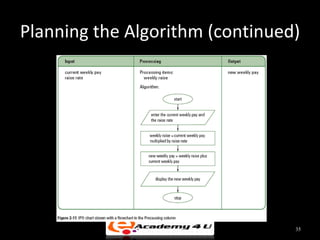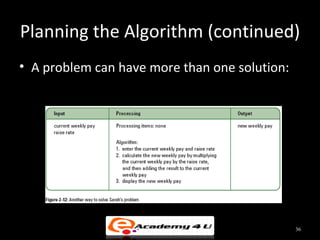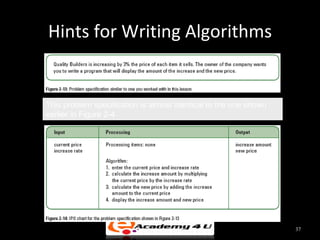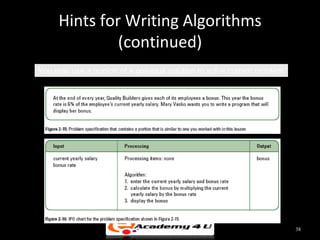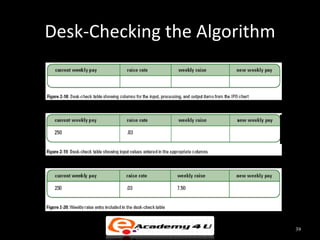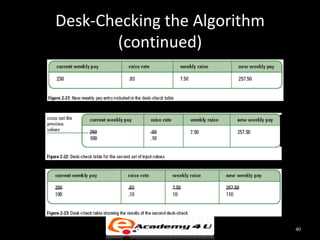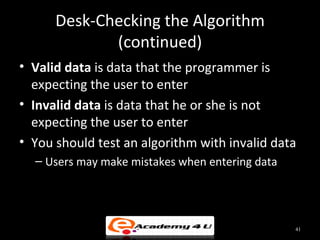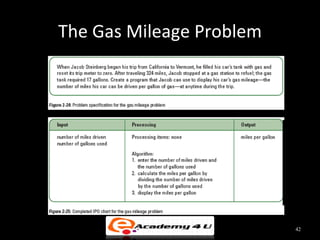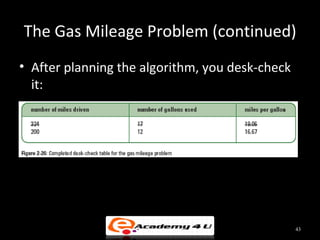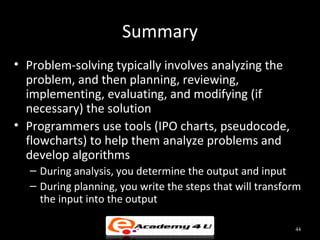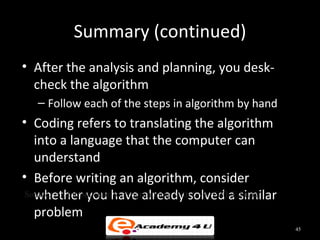1 sur 45
Publicité

### Introduction to problem solving in c++

1. INTRODUCTION TO PROBLEM SOLVING 1
2. Introduction • Algorithm – A sequence of precise instructions that leads to a solution • Program – An algorithm expressed in a language the computer can understand 2
3. Introduction (cont.) Example: 3
4. Introduction (cont.) • Programming is a creative process – No complete set of rules for creating a program 4
5. Programming Process • Program Design Process – Problem Solving Phase • Result is an algorithm that solves the problem – Implementation Phase • Result is the algorithm translated into a programming language • Be certain the task is completely specified – What is the input? – What information is in the output? – How is the output organized? 5
6. Programming Process (cont.) • Develop the algorithm before implementation – Experience shows this saves time in getting your program to run. – Test the algorithm for correctness 6
7. Programming Process (cont.) Implementation Phase • Translate the algorithm into a programming language • Compile the source code – Locates errors in using the programming language • Run the program on sample data – Verify correctness of results • Results may require modification of the algorithm and program 7
8. Programming Process (cont.) 8
9. Programming Process (cont.) Example: • STEP 1: PROBLEM ANALYSIS – Purpose: • To describe in details a solution to a problem by providing the needed information for the problem. – How? • First, understand & study the problem. – Identify: • The input to the problem. • The required output. • The relevant process. For example, scientific formula or appropriate theories if any. 9
10. Programming Process (cont.) Problem : Write a program that get 3 numbers as input from user. Find the average and display the numbers and the average. Problem Analysis: Input: 3 numbers. Process: 1. Add the numbers 2. Divide the total with 3 Output: The 3 numbers and average 10
11. Programming Process (cont.) INPUT PROCESS OUTPUT 11
12. Programming Process - Representation Method • STEP 2: PROGRAM DESIGN • Definition: It defines the framework or the flow or the problem solution 1. Algorithm – Algorithm is a sequence of instructions to solve a problem written in human language and problem can be solved if follow the correct procedure – A programmer writes the solution in the form of an algorithm before coding it into computer language. 12
13. Programming Process - Representation Method (cont.) Example of algorithm to calculate the average of 3 numbers: 1. Set Total=0, average=0; 2. Input 3 numbers 3. Total up the 3 numbers Total= total of 3 numbers 4. Calculate average average=Total/3 5. Display 3 numbers and the average 13
14. Programming Process - Representation Method (cont.) 2. Flowchart • A graphical representation of data, information and process or an orderly step- by-step solution to a problem. 14
15. Programming Process - Representation Method (cont.) Start Example: Input a, b, c Total = a + b + c average = Total / 3 Display a, b, c Display average End 15
16. Programming Process - Representation Method (cont.) 3. Pseudocode • Steps in problem solving written in certain of programming code and certain in human language. • For example, some part use C++ language code and some part use English-like phrases. 16
17. Programming Process - Representation Method (cont.) START INPUT a, b, c Total = a + b + c average = Total / 3 OUTPUT a, b, c OUTPUT average END 17
18. Programming Process - Representation Method (cont.) STEPS 3: PROGRAM CODING • Definition: Write a solution into a specific programming language such as C, C++, COBOL and etc. • Solution: Instructions before it is coded into programming language. • Purpose: To produce a program to develop a system. 18
19. Solving Everyday Problems • First step in solving a problem: analyze it – E.g., problem of being hungry • Next, you plan, review, implement, evaluate, and modify (if necessary) the solution – E.g., if you are still hungry 19
20. Solving Everyday Problems (continued) 20
21. Solving Everyday Problems (continued) 21
22. Creating Computer Solutions to Problems • Analysis tools: IPO charts, pseudocode, flowcharts • To desk-check or hand-trace, use pencil, paper, and sample data to walk through algorithm • A coded algorithm is called a program 22
23. Creating Computer Solutions to Problems (continued) 23
24. Analyzing the Problem • Analyze a problem to: – Determine the goal of solving it • Output – Determine the items needed to achieve that goal • Input • Always search first for the output 24
25. Analyzing the Problem (continued) 25
26. IPO Charts • Use an IPO chart to organize and summarize the results of a problem analysis – IPO: Input, Processing, and Output 26
27. IPO Charts (continued) 27
28. IPO Charts (continued) 28
29. Analyzing the Problem (continued) • First, reduce the amount of information you need to consider in your analysis: 29
30. Analyzing the Problem (continued) • Worse than having too much information is not having enough information to solve problem: 30
31. Analyzing the Problem (continued) • Distinguish between information that is missing and information that is implied: 31
32. Planning the Algorithm • Algorithm: set of instructions that will transform the problem’s input into its output – Record it in the Processing column of the IPO chart • Processing item: intermediate value used by algorithm when processing input into output • Pseudocode is a tool programmers use to help them plan an algorithm – Short English statements 32
33. Planning the Algorithm (continued) 33
34. Planning the Algorithm (continued) • Flowcharts are also used to plan an algorithm – Use standardized symbols – Symbols connected with flowlines – Oval: start/stop symbol – Rectangle: process symbol • Represents tasks such as calculations – Parallelogram: input/output symbol • Represents I/O tasks 34
35. Planning the Algorithm (continued) 35
36. Planning the Algorithm (continued) • A problem can have more than one solution: 36
37. Hints for Writing Algorithms This problem specification is almost identical to the one shown earlier in Figure 2-4 37
38. Hints for Writing Algorithms (continued) You may use a portion of a previous solution to solve current problem 38
39. Desk-Checking the Algorithm 39
40. Desk-Checking the Algorithm (continued) 40
41. Desk-Checking the Algorithm (continued) • Valid data is data that the programmer is expecting the user to enter • Invalid data is data that he or she is not expecting the user to enter • You should test an algorithm with invalid data – Users may make mistakes when entering data 41
42. The Gas Mileage Problem 42
43. The Gas Mileage Problem (continued) • After planning the algorithm, you desk-check it: 43
44. Summary • Problem-solving typically involves analyzing the problem, and then planning, reviewing, implementing, evaluating, and modifying (if necessary) the solution • Programmers use tools (IPO charts, pseudocode, flowcharts) to help them analyze problems and develop algorithms – During analysis, you determine the output and input – During planning, you write the steps that will transform the input into the output 44
45. Summary (continued) • After the analysis and planning, you desk- check the algorithm – Follow each of the steps in algorithm by hand • Coding refers to translating the algorithm into a language that the computer can understand • Before writing an algorithm, consider Source: An Introductionhave already solved a similar whether you to Programming with C++, Fifth Edition problem 45
Publicité